Most Affordable JEE | NEET | 8,9,10 Preparation by Kota's Top IITian Doctor Faculties

# General Organic Chemistry - JEE Main Previous Year Questions with Solutions`
JEE Main Previous Year Papers Questions of Chemistry With Solutions are available at eSaral. Simulator   Previous Years AIEEE/JEE Mains Questions
Q. Arrange the carbanions, $\left(\mathrm{CH}_{3}\right)_{3} \overline{\mathrm{C}}, \overline{\mathrm{C}} \mathrm{Cl}_{3},\left(\mathrm{CH}_{3}\right)_{2} \overline{\mathrm{C}} \mathrm{H}, \mathrm{C}_{6} \mathrm{H}_{5} \overline{\mathrm{C}} \mathrm{H}_{2}$ , in order of their decreasing stability [AIEEE-2009]
Ans. (1)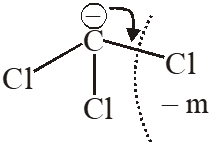Q. The non aromatic compound among the following is :-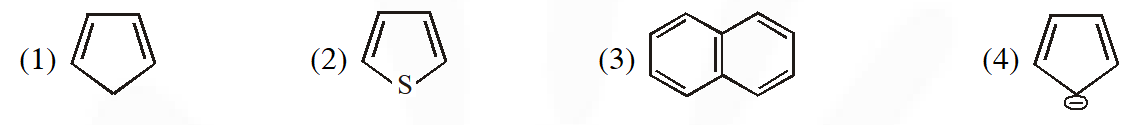[AIEEE-2011]
Ans. (1)
Q. ortho-Nitrophenol is less soluble in water than p– and m– Nitrophenols because :- (1) Melting point of o–Nitrophenol is lower than those of m– and p– isomers (2) o–Nitrophenol is more volatile in steam than those of m– and p– isomers (3) o–Nitrophenol shows Intramolecular H–bonding (4) o–Nitrophenol shows Intermolecular H–bonding [AIEEE-2012]
Ans. (3)
Q. Which of the following compounds are antiaromatic :-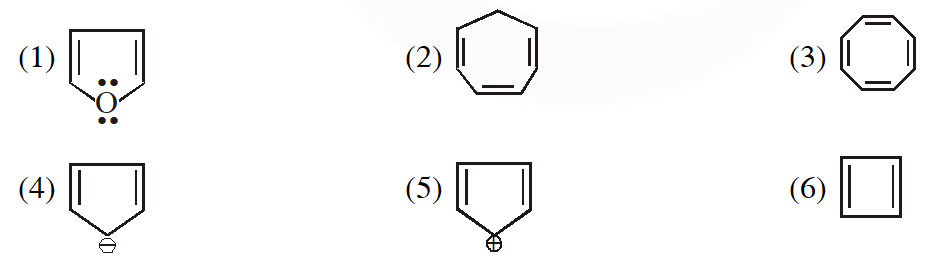(1) (1) and (6) (2) (2) and (5) (3) (1) and (5) (4) (5) and (6) [AIEEE-2012(Online)]
Ans. (4)
Q. Among the following the molecule with the lowest dipole moment is :- (1) $\mathrm{CHCl}_{3}$ (2) $\mathrm{CH}_{2} \mathrm{Cl}_{2}$ (3) $\mathrm{CCl}_{4}$ (4) $\mathrm{CH}_{3} \mathrm{Cl}$ [AIEEE-2012(Online)]
Ans. (3)
Q. The order of stability of the following carbocations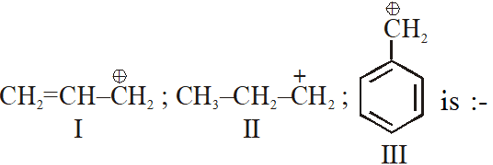(1) III > II > I (2) II > III > I (3) I > II > III (4) III > I > II [JEE-MAIN-2013]
Ans. (4)
Q. For which of the following molecule significant $\mu^{1} 0$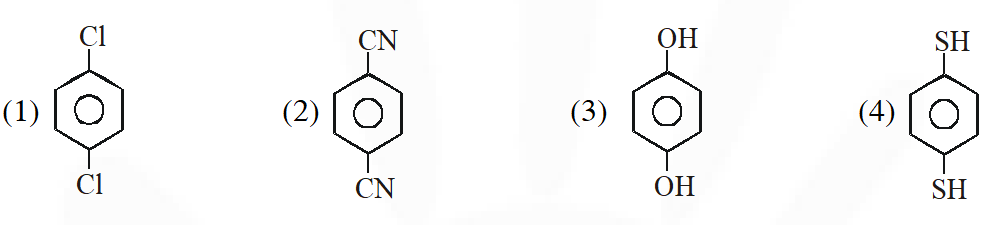(1) Only (3) (2) (3) and (4) (3) Only (1) (4) (1) and (2) [JEE-MAIN-2014]
Ans. (2)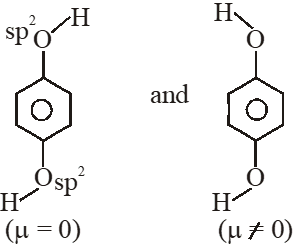So dipole moment per mole (containing $\mathrm{N}_{\mathrm{A}}$ molecules) is non zero. Some concept applicable for option (4) .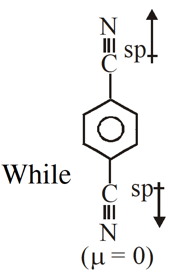Q. The correct order of increasing basicity of the given conjugate base $\left(\mathrm{R}=\mathrm{CH}_{3}\right.$) is :- $(1) \mathrm{RCO} \overline{\mathrm{O}}<\mathrm{HC} \equiv \overline{\mathrm{C}}<\overline{\mathrm{N}} \mathrm{H}_{2}<\overline{\mathrm{R}}$ (2) $\mathrm{RCO} \overline{\mathrm{O}}<\mathrm{HC} \equiv \overline{\mathrm{C}}<\overline{\mathrm{R}}<\overline{\mathrm{N}} \mathrm{H}_{2}$ (3) $\quad \overline{\mathrm{R}}<\mathrm{HC} \equiv \overline{\mathrm{C}}<\mathrm{RCO} \overline{\mathrm{O}}<\overline{\mathrm{N}} \mathrm{H}_{2}$ (4) $\mathrm{RCO} \overline{\mathrm{O}}<\overline{\mathrm{N} H}_{2}<\mathrm{HC} \equiv \overline{\mathrm{C}}<\overline{\mathrm{R}}$ [AIEEE-2010]
Ans. (1) Basic strength $\propto \frac{1}{\text { Stability of conjugate base }}$
Q. The strongest acid amongst the following compounds is ? (1) $\mathrm{CH}_{3} \mathrm{CH}_{2} \mathrm{CH}(\mathrm{Cl}) \mathrm{CO}_{2} \mathrm{H}$ (2) $\mathrm{ClCH}_{2} \mathrm{CH}_{2} \mathrm{CH}_{2} \mathrm{COOH}$ (3) $\mathrm{CH}_{3} \mathrm{COOH}$ (4) HCOOH [AIEEE-2011]
Ans. (1) (Acidity a –I effect)
Q. The correct order of acid strength of the following compounds :- A. Phenol B. p-Cresol C. m-Nitrophenol D. p- Nitrophenol (1) C > B > A > D (2) D > C > A > B (3) B > D > A > C (4) A > B > D > C [AIEEE-2011]
Ans. (2)
Q. In the following compounds :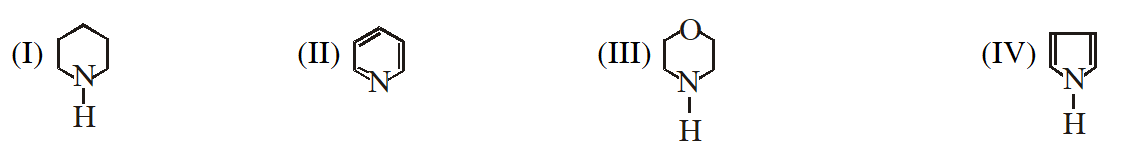the order of basicity is as follows : (1) IV > III > II > I (2) II > III > I > IV (3) I > III > II > IV (4) III > I > II > IV [JEE(Main)-2012]
Ans. (3)
Q. The most basic compound among the following is :- (1) Acetanilide (2) Benzylamine (3) p-Nitro aniline (4) Aniline [JEE(Main)-2012]
Ans. (2) Basicity $\propto \frac{1}{\text { Resonance of lone pair e }}$
Q. The order of basicity of amines in gaseous state is :- (1) $3^{\circ}>2^{\circ}>\mathrm{NH}_{3}>1^{\circ}$ (2) $1^{\circ}>2^{\circ}>3^{\circ}>\mathrm{NH}_{3}$ (3) $\mathrm{NH}_{3}>1^{\circ}>2^{\circ}>3^{\circ}$ (4) $3^{\circ}>2^{\circ}>1^{\circ}>\mathrm{NH}_{3}$ [JEE(Main)-2013]
Ans. (4) Basicity $\propto+I$ effect
Q. Arrange the following compounds in order of decreasing acidity :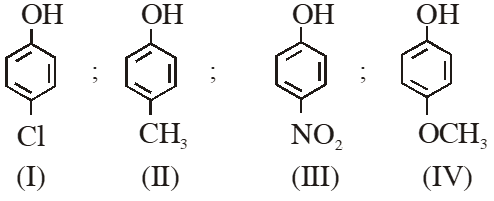(1) II > IV > I > III (2) I > II > III > IV (3) III > I > II > IV (4) IV > III > I > II [JEE(Main)-2013]
Ans. (3) Acidity $\propto-\mathrm{I} /-\mathrm{R} \propto \frac{1}{+\mathrm{I} /+\mathrm{R}}$
Q. The conjugate base of hydrazoic acid is :- (1) $\mathrm{HN}_{3}^{-}$ (2) $\mathrm{N}_{3}^{-}$ (3) $\mathrm{N}_{2}^{-}$ (4) $\mathrm{N}^{-3}$ [JEE(Main)-2014]
Ans. (2) $\left(\mathrm{HN}_{3} \longrightarrow \mathrm{N}_{3}+\mathrm{H}^{+}\right)$
Q. Which one of the following compounds will not be soluble in sodium bicarbonate ? (1) Benzene sulphonic acid (2) Benzoic acid (3) o-Nitrophenol (4) 2, 4, 6 - Trinitrophenol [JEE(Main)-2014]
Ans. (2) Due to ortho effect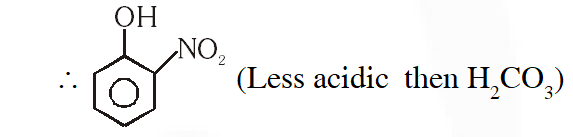Q. Considering the basic strength of amines in aqueous solution, which one has the smallest $\mathrm{pK}_{\mathrm{b}}$ value ? (1) $\left(\mathrm{CH}_{3}\right)_{3} \mathrm{N}$ (2) $\mathrm{C}_{6} \mathrm{H}_{5} \mathrm{NH}_{2}$ (3) $\left(\mathrm{CH}_{3}\right)_{2} \mathrm{NH}$ (4) $\mathrm{CH}_{3} \mathrm{NH}_{2}$ [JEE(Main)-2014]
Ans. (3) \begin{aligned} \therefore \text { Due to solvation and inductive effect } \\ 2^{\circ}>1^{\circ}>3^{\circ}>0^{\circ} & \mathrm{R}=-\mathrm{CH}_{3} \end{aligned}
Q. Among the following oxoacids, the correct decreasing order of acid strength is : (1) $\mathrm{HClO}_{4}>\mathrm{HClO}_{3}>\mathrm{HClO}_{2}>\mathrm{HOCl}$ (2) $\mathrm{HClO}_{2}>\mathrm{HClO}_{4}>\mathrm{HClO}_{3}>\mathrm{HOCl}$ (3) $\mathrm{HOCl}>\mathrm{HClO}_{2}>\mathrm{HClO}_{3}>\mathrm{HClO}_{4}$ (4) $\mathrm{HClO}_{4}>\mathrm{HOCl}>\mathrm{HClO}_{2}>\mathrm{HClO}_{3}$ [JEE(Main)-2014]
Ans. (1) $\therefore$ Acidic $\propto$ more E.R.S. of conjugate base
Q. The increasing order of basicity of the following compounds is :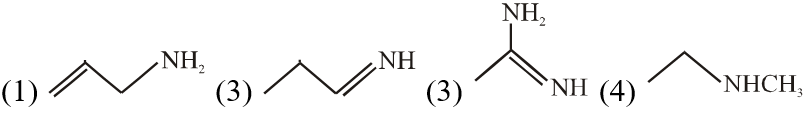(1) (1) < (1) < (3) < (4) (2) (1) < (1) < (4) < (3) (3) (4) < (2) < (1) < (3) (4) (1) < (2) < (3) < (3) [JEE(Main)-2018]
Ans. (2) Order of base nature depends on electron donation tendency. In compound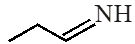nitrogen is sp2 hybridized so least basic among all given compound. compound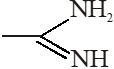is very strong nitrogeneous organic base as lone pair of one nitrogen delocalize in resonance and make another nitrogen negativly charged and conjugate acid have two equivalent resonating structure. Thus it is most basic in given compouds.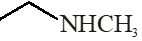(secondary amine) more basic than(primary amine)

Sept. 8, 2023, 11:14 a.m.
Great 👍
Om ganesh amale
April 1, 2023, 6:35 a.m.
This is important for jee mains
Sonu keshri
Feb. 24, 2023, 7:28 p.m.
Thank u 😊😊
Arman
Jan. 4, 2022, 10:05 p.m.
This is important for neet
Arun Prakash
Sept. 4, 2023, 9:08 p.m.
Yes
Hemarao
Dec. 29, 2021, 4:20 p.m.
Nice
Sathyam
Dec. 29, 2021, 4:20 p.m.
Nice
sunny
Nov. 25, 2021, 3:16 p.m.
this is very useful which helps in jee mains
VBR SHARMA
Aug. 9, 2021, 10:52 p.m.
THIS IS VERY VERY USEFUL WHICH HELPS IN JEE MAINS ADVANCED
kayra sharma
May 17, 2021, 9:54 a.m.
thanks a lot
keerthana
April 28, 2021, 3:49 p.m.
excellent
Sunil Kumar Mishra
March 20, 2021, 9:15 a.m.
Best
Shiva
March 13, 2021, 5:24 p.m.
Good
tanvir
March 11, 2021, 9:20 p.m.
great good
esaral
March 11, 2021, 9:19 p.m.
great
March 3, 2021, 4:33 p.m.
Comment
Feb. 23, 2021, 7:10 p.m.
Awsome helps a lot
kakarot
Feb. 21, 2021, 5:47 p.m.
good site!!!
Aashika sharma
Feb. 21, 2021, 12:47 a.m.
Esaral is always veey good for jee aspirant
xyz
Feb. 18, 2021, 10:02 p.m.
answers options are usually written wrong while in explanation the options are right
Feb. 16, 2021, 9 a.m.
Thank u
student
Feb. 15, 2021, 9:16 p.m.
nice content
Anonymous
Dec. 30, 2020, 9:10 a.m.
so many errors
anonymous
Dec. 22, 2020, 9:55 a.m.
many errors in the questions
Sunny Patel
Dec. 17, 2020, 11:32 p.m.
Thanks
Amir
Dec. 9, 2020, 9:29 p.m.
thanx
Good but Last one ........ you copied but yet wrong.
Nov. 19, 2020, 6:28 p.m.
Anonymous
Harshita
Nov. 2, 2020, 1:06 a.m.
There are good questions but many errors like some question are not in this that ask in jee main but still it is very helpful to me👍👍
ERROR
Oct. 17, 2020, 12:54 p.m.
too many errors
Atharva Kamble
Oct. 16, 2020, 12:58 p.m.
Best quality questions thanks to esaral
Akshar
Sept. 18, 2020, 10:08 p.m.
Last mcq me galti hai !!!! Thank for everything
gowthami
Aug. 31, 2020, 11:55 a.m.
tnq
alok kumar gothwal
Aug. 26, 2020, 10:25 a.m.
very good contect,bette if there is latest question i.e 2019&amp;2020 (january shift)
Gayathri
Aug. 24, 2020, 11:32 a.m.
Very good content ,better if there is latest questions i.e 2019and2020(January shift)
Chanukya
Aug. 22, 2020, 7:25 p.m.
So it is well understanding explanation but we can only do this if the concept is clear and you should add exp
Sriya
Aug. 20, 2020, 9:03 a.m.
Aug. 10, 2020, 6:27 a.m.
the strategy of questions without visibility of direct anwers there is so.....good,but it would be still better if remaining questions are added to this list
Aug. 9, 2020, 12:09 p.m.
July 27, 2020, 8:49 a.m.
Nice it is interesting to do some more questions
Samiran Dilipkumar Kulkarni
July 21, 2020, 10:23 a.m.
Really very good 😀😀😀
student
June 27, 2020, 3:44 p.m.
all questions are not available.it would be better for the students if you add remaining questions also
June 27, 2020, 3:14 p.m.
There are little errors in the solutions Please correct it
Garima
June 22, 2020, 3:49 p.m.
Include more que. Cover all Concept
Panku
June 17, 2020, 8:56 p.m.
Super'b but how can I get PDF of this question😁
June 15, 2020, 9:53 a.m.
Good enough ...But sometimes show solution does not work... Please try to fix it.👍
Anonymous Student
June 7, 2020, 10:29 p.m.
can I get a PDF of these questions? Kindly let me know ASAP!
Ravva
May 27, 2020, 9:55 a.m.
Thopp